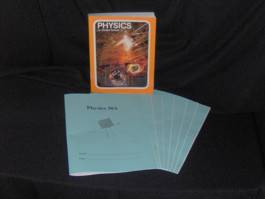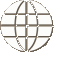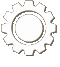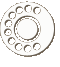COURSE MaterialPhysics 30 (Grade 12)

Physics 30, designed to satisfy the objectives of Saskatchewan Learning’s Physics 30 course, is written from a Biblical perspective and is divided into eight units. The required resource for the course is Physics for Christian Schools, by Rosemary A. Lasell and Paul Wilt, Bob Jones University Press.

This workbook series was developed for use by schools using an individualized, mastery-based learning system, and therefore comes with score keys, tests, and test keys.

Upon completion of each unit, the student should be able to:

Unit 1

• understand the difference between kinematics and dynamics
• state the three equations of motion
• solve speed acceleration problems
• solve problems with objects in free fall
• derive needed information from position/time or velocity time graphs

Unit 2

• add and subtract vectors
• determine the missing parts of a triangle using Pythagorean’s Theorem
• calculate the displacement of an object moving in two dimensions
• determine the velocity of an object moving in two dimensions
• determine the acceleration of an object moving in two dimensions
• apply the equations of motion to an object moving in two dimensions

Unit 3

• understand balanced and unbalanced forces
• recognize Newton’s three laws of motion and apply the laws to solve problems
• distinguish between mass and weight
• draw free-body diagrams
• understand normal, transmitted, and frictional forces

Unit 4

• describe circular motion
• solve problems in circular motion applying Newton’s laws and the equations for circular motion
• apply the law of universal gravitation to calculate the force between two objects

Unit 5

• solve problems involving work, power, and energy
• distinguish between kinetic and potential energy
• understand the law of conservation of energy and apply this law to solve problems
• determine whether total mechanical energy is conserved or not

Unit 6

• understand momentum and solve problems involving momentum
• determine what causes a change in momentum
• understand impulse
• determine when momentum is conserved
• apply the conservation law to solve problems
• solve problems involving elastic and inelastic collisions

Unit 7

• understand electric charges
• recognize and understand electric fields and apply Coulomb’s law to calculate the force exerted by an electric field
• understand how electric charge is stored by a capacitor
• identify the factors in resistance
• recognize and understand Ohm’s law and Joule’s law, and apply them to solve problems
• solve simple circuits
• distinguish between series and parallel circuits

Unit 8

• define the types of natural radioactivity
• describe nuclear fission describe the operation of the CANDU nuclear reactor
COURSE MaterialsSAICS has developed Christian curriculum (where none other was available) to match Saskatchewan Learning objectives for high school courses.

View Our Course Listing
ORDERING CoursesFind out more information about ordering courses that have been developed by SAICS including score keys and associated textbooks.

Ordering Information